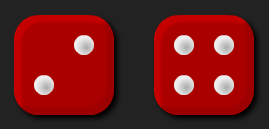#### You may also like### Chocolate

There are three tables in a room with blocks of chocolate on each. Where would be the best place for each child in the class to sit if they came in one at a time?### Four Triangles Puzzle

Cut four triangles from a square as shown in the picture. How many different shapes can you make by fitting the four triangles back together?### Cut it Out

Can you dissect an equilateral triangle into 6 smaller ones? What number of smaller equilateral triangles is it NOT possible to dissect a larger equilateral triangle into?

# Round the Dice Decimals 1

## Round the Dice Decimals 1There are two dice, each of them with faces labelled from 1 to 6.
When the dice are rolled they can be combined in two different ways to make a number less than 10 with one decimal place.

For example, if I roll a 2 and a 3 I can combine them to make 2.3 or 3.2.

Now round each of these numbers to the nearest whole number: 2.3 rounds to 2 and 3.2 rounds to 3. Repeat for other rolls of the dice.

Do both of the numbers you make ever round to the same whole number?

There some interactive dice here that you can use for this problem.

#### Why do this activity?

This activity provides a meaningful task for practising rounding decimal numbers to the nearest whole number. It encourages children to record their results, notice patterns and make predictions.

#### Possible approach

A possible starting point is using a number line to remind the class what is meant by rounding.
The interactive dice can be used to model the activity on an interactive whiteboard or can be used by the children as they engage with the task. You may also find that individual dice are useful.

As the task is being modelled, the results should be recorded in a table, perhaps like the one below:

 Numbers rolled 1st decimal number Rounds to 2nd decimal number Rounds to 2 and 3 2.3 2 3.2 3 2 and 6 2.6 3 6.2 6

This organisation of results will support the children to notice patterns and conjecture about when numbers will round to different whole numbers.

Some children may move onto the extension tasks (below).

#### Key questions

Which numbers can we make?
What will they round to? Will they round up or down? Why?
Do they round to the same whole number? Why? When will this happen?
Does it make a difference if the digits rolled are unique?

#### Possible extensions

Extension 1: Did the class find examples where both decimal numbers round to the same whole number? Can they then come up with a rule about when both of the decimal numbers will round to the same whole number?
Extension 2: Having completed the original task, ask the children to add a column to the right hand side of their table to note when numbers round up or down. Can they predict from the initial dice roll, whether the decimal numbers made will round up or down?
Extension 3: What if you change the numbers on the faces of the dice?
Extension 4: What happens if you introduce a third dice and make six different decimal numbers less than 10? Can they ever all round to the same whole number?
Extension 5: Have a go at the activity Round the Dice Decimals 2 and practise rounding numbers with two decimal places.

#### Possible support

Copies of the table to write straight into would be useful.
A number line would also be handy to support children in reasoning about which whole number is closest to a given decimal number.